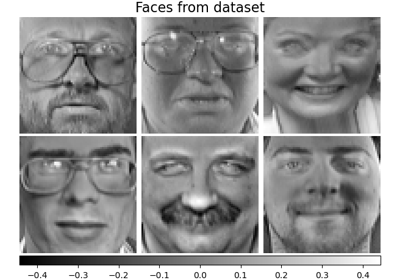# sklearn.decomposition.SparsePCA¶

class sklearn.decomposition.SparsePCA(n_components=None, *, alpha=1, ridge_alpha=0.01, max_iter=1000, tol=1e-08, method='lars', n_jobs=None, U_init=None, V_init=None, verbose=False, random_state=None)[source]

Sparse Principal Components Analysis (SparsePCA).

Finds the set of sparse components that can optimally reconstruct the data. The amount of sparseness is controllable by the coefficient of the L1 penalty, given by the parameter alpha.

Read more in the User Guide.

Parameters:
n_componentsint, default=None

Number of sparse atoms to extract. If None, then n_components is set to n_features.

alphafloat, default=1

Sparsity controlling parameter. Higher values lead to sparser components.

ridge_alphafloat, default=0.01

Amount of ridge shrinkage to apply in order to improve conditioning when calling the transform method.

max_iterint, default=1000

Maximum number of iterations to perform.

tolfloat, default=1e-8

Tolerance for the stopping condition.

method{‘lars’, ‘cd’}, default=’lars’

Method to be used for optimization. lars: uses the least angle regression method to solve the lasso problem (linear_model.lars_path) cd: uses the coordinate descent method to compute the Lasso solution (linear_model.Lasso). Lars will be faster if the estimated components are sparse.

n_jobsint, default=None

Number of parallel jobs to run. None means 1 unless in a joblib.parallel_backend context. -1 means using all processors. See Glossary for more details.

U_initndarray of shape (n_samples, n_components), default=None

Initial values for the loadings for warm restart scenarios. Only used if U_init and V_init are not None.

V_initndarray of shape (n_components, n_features), default=None

Initial values for the components for warm restart scenarios. Only used if U_init and V_init are not None.

verboseint or bool, default=False

Controls the verbosity; the higher, the more messages. Defaults to 0.

random_stateint, RandomState instance or None, default=None

Used during dictionary learning. Pass an int for reproducible results across multiple function calls. See Glossary.

Attributes:
components_ndarray of shape (n_components, n_features)

Sparse components extracted from the data.

error_ndarray

Vector of errors at each iteration.

n_components_int

Estimated number of components.

New in version 0.23.

n_iter_int

Number of iterations run.

mean_ndarray of shape (n_features,)

Per-feature empirical mean, estimated from the training set. Equal to X.mean(axis=0).

n_features_in_int

Number of features seen during fit.

New in version 0.24.

feature_names_in_ndarray of shape (n_features_in_,)

Names of features seen during fit. Defined only when X has feature names that are all strings.

New in version 1.0.

PCA

Principal Component Analysis implementation.

MiniBatchSparsePCA

Mini batch variant of SparsePCA that is faster but less accurate.

DictionaryLearning

Generic dictionary learning problem using a sparse code.

Examples

>>> import numpy as np
>>> from sklearn.datasets import make_friedman1
>>> from sklearn.decomposition import SparsePCA
>>> X, _ = make_friedman1(n_samples=200, n_features=30, random_state=0)
>>> transformer = SparsePCA(n_components=5, random_state=0)
>>> transformer.fit(X)
SparsePCA(...)
>>> X_transformed = transformer.transform(X)
>>> X_transformed.shape
(200, 5)
>>> # most values in the components_ are zero (sparsity)
>>> np.mean(transformer.components_ == 0)
0.9666...


Methods

 fit(X[, y]) Fit the model from data in X. fit_transform(X[, y]) Fit to data, then transform it. get_feature_names_out([input_features]) Get output feature names for transformation. Get metadata routing of this object. get_params([deep]) Get parameters for this estimator. Transform data from the latent space to the original space. set_output(*[, transform]) Set output container. set_params(**params) Set the parameters of this estimator. Least Squares projection of the data onto the sparse components.
fit(X, y=None)[source]

Fit the model from data in X.

Parameters:
Xarray-like of shape (n_samples, n_features)

Training vector, where n_samples is the number of samples and n_features is the number of features.

yIgnored

Not used, present here for API consistency by convention.

Returns:
selfobject

Returns the instance itself.

fit_transform(X, y=None, **fit_params)[source]

Fit to data, then transform it.

Fits transformer to X and y with optional parameters fit_params and returns a transformed version of X.

Parameters:
Xarray-like of shape (n_samples, n_features)

Input samples.

yarray-like of shape (n_samples,) or (n_samples, n_outputs), default=None

Target values (None for unsupervised transformations).

**fit_paramsdict

Returns:
X_newndarray array of shape (n_samples, n_features_new)

Transformed array.

get_feature_names_out(input_features=None)[source]

Get output feature names for transformation.

The feature names out will prefixed by the lowercased class name. For example, if the transformer outputs 3 features, then the feature names out are: ["class_name0", "class_name1", "class_name2"].

Parameters:
input_featuresarray-like of str or None, default=None

Only used to validate feature names with the names seen in fit.

Returns:
feature_names_outndarray of str objects

Transformed feature names.

Get metadata routing of this object.

Please check User Guide on how the routing mechanism works.

Returns:

A MetadataRequest encapsulating routing information.

get_params(deep=True)[source]

Get parameters for this estimator.

Parameters:
deepbool, default=True

If True, will return the parameters for this estimator and contained subobjects that are estimators.

Returns:
paramsdict

Parameter names mapped to their values.

inverse_transform(X)[source]

Transform data from the latent space to the original space.

This inversion is an approximation due to the loss of information induced by the forward decomposition.

New in version 1.2.

Parameters:
Xndarray of shape (n_samples, n_components)

Data in the latent space.

Returns:
X_originalndarray of shape (n_samples, n_features)

Reconstructed data in the original space.

set_output(*, transform=None)[source]

Set output container.

See Introducing the set_output API for an example on how to use the API.

Parameters:
transform{“default”, “pandas”}, default=None

Configure output of transform and fit_transform.

• "default": Default output format of a transformer

• "pandas": DataFrame output

• None: Transform configuration is unchanged

Returns:
selfestimator instance

Estimator instance.

set_params(**params)[source]

Set the parameters of this estimator.

The method works on simple estimators as well as on nested objects (such as Pipeline). The latter have parameters of the form <component>__<parameter> so that it’s possible to update each component of a nested object.

Parameters:
**paramsdict

Estimator parameters.

Returns:
selfestimator instance

Estimator instance.

transform(X)[source]

Least Squares projection of the data onto the sparse components.

To avoid instability issues in case the system is under-determined, regularization can be applied (Ridge regression) via the ridge_alpha parameter.

Note that Sparse PCA components orthogonality is not enforced as in PCA hence one cannot use a simple linear projection.

Parameters:
Xndarray of shape (n_samples, n_features)

Test data to be transformed, must have the same number of features as the data used to train the model.

Returns:
X_newndarray of shape (n_samples, n_components)

Transformed data.

## Examples using sklearn.decomposition.SparsePCA¶Faces dataset decompositions

Faces dataset decompositions